# Mueller Matrices for Polarizing Elements

### What Are Mueller Matrices?

Mueller matrices are 4x4 matrices that describe the linear relationship between polarization states of the incident light beam and the emerging light beam after passing through some polarizing elements, i.e., polarizers, waveplates, rotators.

Mueller matrices are used together with Stokes parameters (Stokes vectors). Please check out this article if you need a review of Stokes parameters.

Now let's look at figure 1 below.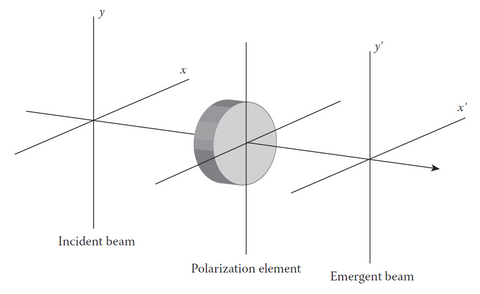Figure 1: Interaction of a polarized beam with a polarizing element

The incident beam is characterized by its Stokes parameters S:The incident beam interacts with the polarizing element, and the emerging light beam has its Stokes parameters S':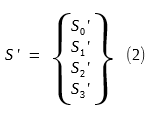In Mueller matrices, we only consider a linear relationship between S and S', thus S' can be expressed as a linear combination of the four Stokes parameters of the incident beam:In matrix form, we can write these equations as: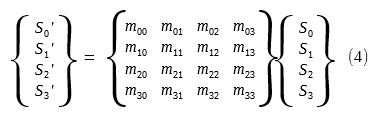orM is the 4x4 matrix known as the Mueller matrix.

Hans Mueller was the first to describe polarizing components, that is, polarizers (also called diattenuators), phase shifters (also called waveplate, retarder), rotators, depolarizers, in terms of Mueller matrices.

### What Are Polarizing Elements?

When light beam interacts with matter, its polarization state is almost always changed. There are four types of changes:

• The amplitudes of its orthogonal components (x, y)
• The relative phase between its orthogonal components (x, y)
• The vibration directions of its orthogonal components (x, y)
• Energy transfer from polarized states to unpolarized states

There are mainly four types of polarizing elements:

• Polarizers (also called diattenuators) - that change the orthogonal amplitudes (x, y) unequally
• Phase Shifters (also called waveplates, retarders) - that add a phase shift between the orthogonal components (x, y)
• Rotators - that rotate the orthogonal components (x, y) equally through an angle θ (such as Faraday rotators)
• Depolarizers - that scramble the polarization states and produce pseudo-random output polarizations. (transfer energy from polarized states to unpolarized states)

### Mueller Matrix of a Linear Polarizer

A polarizer is actually an anisotropic attenuator that attenuates the orthogonal components of an light beam unequally.

As shown in figure 2 below, a polarized incident light beam passes through a polarizer. We now discuss the polarization states change made by the polarizer.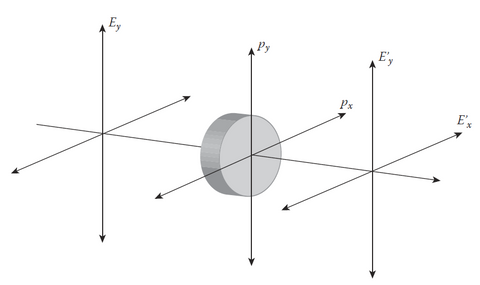Figure 2: A linear polarizer with attenuation coefficients px and py

The orthogonal components of the incident light beam are represented by its complex amplitudes Ex and Ey respectively.

Similarly, the orthogonal components of the emerging light beam are represented by Ex' and Ey'.

The relationship between the incident light beam and the emerging light beam are described by two attenuation coefficients px and py :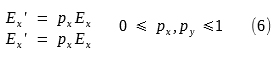For no attenuation along an axis px (or py ) = 1, for completely attenuation along an axis px (or py ) = 0. The no attenuation axis is called the transmission axis.

Stokes Parameters

The Stokes parameters S (incident beam) and S' (emerging beam) as expressed in complex form are: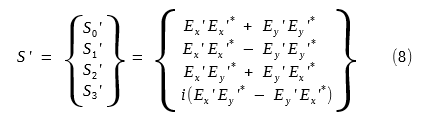By substituting (6) into (8), and using (7), we then get: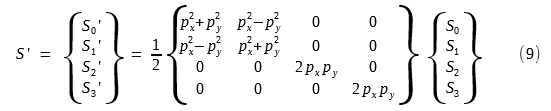Mueller Matrix

Thus, the Mueller matrix for a linear polarizer with amplitude attenuation coefficients px and py is:Note:

px and py are amplitude attenuation coefficients which are NOT directly observable (since lights vibrate very fast at 1015 Hz order, which is too fast for any equipment to detect directly).

px2 and py2 are intensity transmittances that are directly observable. (Stokes parameters are based on intensity measurement).

We can rewrite (10) in terms of trigonometric functions by setting: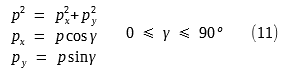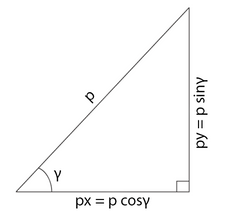We can then rewrite (10) as: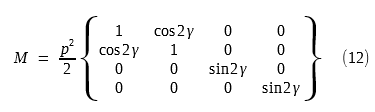Mueller Matrix for Ideal Linear Polarizers

In (12), ideal perfect linear polarizers have p = 1.

Thus, the Mueller matrix for an ideal linear horizontal polarizer is ( γ = 0º ) :And, the Mueller matrix for an ideal linear vertical polarizer is γ = 90º ) :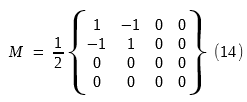Note:

If the original light beam is completely unpolarized, the maximum intensity of the emerging light beam is only 50% of the original intensity. This is the price we pay for obtaining perfectly polarized light.

The only known natural material that comes close to an ideal polarizer is calcite.

### Mueller Matrix of A Phase Shifter (also called Waveplate, Retarder)

As shown in figure 3 below, a phase shifter introduces a phase shift of φ between the orthogonal components of the incident light beam.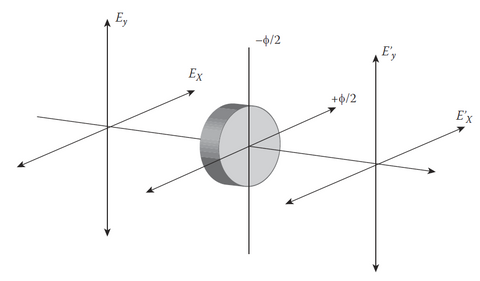Figure 3: A Phase Shifter (Waveplate, Retarder)

The x component gets a +φ/2 phase shift (fast axis), and the y component gets a -φ/2 phase shift (slow axis). Thus, the phase shift between x and y is φ.

Thus, the emerging beam's complex amplitudes are related to the incident beam's amplitudes by:Stokes Parameters

We refer to (7) and (8) for the Stokes parameters of incident beam S and emerging beam S', and substitute (15) into (7), (8). Then we get can the relationship between S' and S as: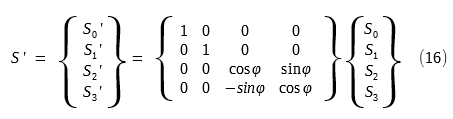Since this is an ideal phase shifter, there is no attenuation at all, thus S0' = S0.

Mueller Matrix for Ideal Phase Shifters

From (16), we get the Mueller matrix for an ideal phase shifter as:There are two most commonly used waveplates (phase shifters):

• Quarter-wave Plate (φ = 90º, y component is delayed by one quarter wavelength than x component)
• Half-wave Plate (φ = 180º, y component is delayed by one half wavelength than x component)

Quarter-wave Plate:

For quarter-wave plate, φ = 90º, thus the Mueller matrix is: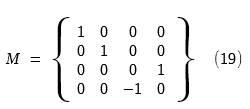Half-wave Plate:

For half-wave plate, φ = 180º, thus the Mueller matrix is: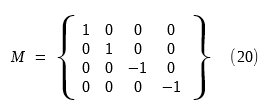### Mueller Matrix of a Rotator

A polarization rotator rotates the orthogonal field components (Ex, Ey) by an angle θ and becomes (Ex', Ey') as shown in figure 4 below.Figure 4: Polarization Rotator

We can project (Ex, Ey) on to Ex' and Ey' axes, and we then get: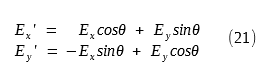Note:

(21) is the amplitude equations for rotation.

In order to find the Mueller matrix (which is based on intensity and Stokes parameters), we make use of (7), (8) and (21), and get the relationship between Stokes parameter S' and S as:Thus, the Mueller matrix for a polarization rotator of angle θ is:Note:

The physical rotation of angle θ on the orthogonal FIELD components (Ex, Ey) leads to in the Mueller matrix.

This is because Mueller matrix is in INTENSITY domain which has a SQUARE relationship with FIELD components (Ex, Ey).

### Mueller Matrix of Rotated Polarizing Elements

In the previous derivations, we have aligned the polarizing elements' axes along the Ex and Ey axes.

But what if the polarizing elements' axes don't align perfectly with Ex and Ey, say, with an angle θ instead?

This is actually a more common practice in real world optical labs. Thus we have to master it.

Let's assume that a polarizing element whose Mueller matrix is M and its x' axis is aligned with an angle θ in respect to x axis of the optical bench set up.

This is shown in figure 5 below.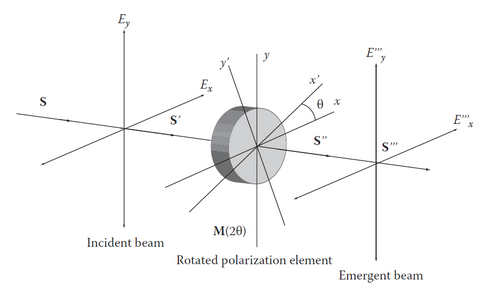The incident light beam has its Stokes parameter S which is based on x-y axes.

Since the polarizing element axes x'-y' has an angle θ in regard to x-y axes, we need to map S into S' which is based on x'-y' axes.

This is done by multiplying S with a Mueller matrix of a polarization rotator of angle θ (as shown in the last section).

As seen from last section, this Mueller matrix is:Thus we have this relationship:S' then incidents on the polarizing element. Since S' is already aligned at x'-y' axes, we can directly multiply S' with this element's Mueller matrix M.

Thus we have: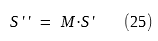Since S'' is aligned at x'-y' axes, we need to rotate it a θ angle to get the final Stokes parameter S''' which is aligned with x-y axes.

Thus we get: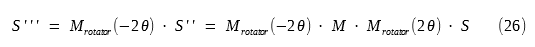We then get the Mueller matrix M(2θ) for the whole system as: